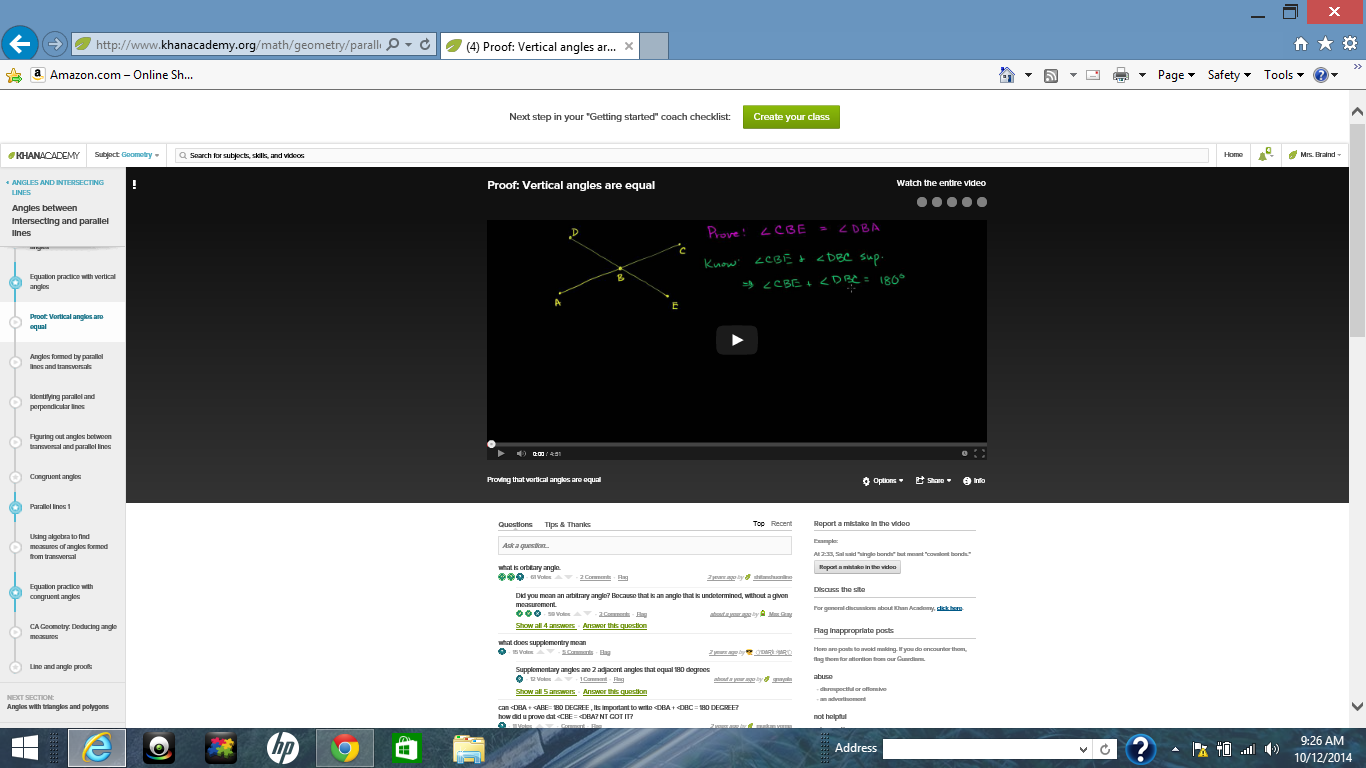Module 5: Circle.  This topic starts on Friday, March 3, 2017

1.     Video: Intro to arc measure

2.     Video:Arc measures

3.     Practice: Arc Measure

4.     Video: Finding arc measures with equations

5.     Practice: Arc measure with equations

6.     Practice: Arc length

8.     Practice: Inscribed angles

9.     Practice: Tangents of circles problems

Geometry analogies

DAY is to  night    as         convex  is to      ___________________________

Tall is to  SHORT   AS       PARALLEL  is to  ________________________

NODE IS TO  GRAPH THEORY  AS   DOT IS TO  ________________________

VAT IS TO  VERTICAL ANGLE THEOREM AS

BAT is to __________________________________________________________________

Ordered point is to  coordinate geometry  as

Location is to  _______________________________________________________

Circle is to sphere as  line is to  ____________________________________

TrIANGLE IS TO  PYRAMID  AS  ELLIPSE IS TO ____________________

QUADRILATERAL IS TO  PRISM AS  LINE IS TO_____________________

## Relationships Between Reflections and Symmetry

Read the information below and then do problems 18-21.  Turn in your work by October 31, 2014.
•
 Bilateral Symmetry   If the image of a shape after reflection is exactly the same as the shape itself, we say the shape has bilateralsymmetry. The mirror line that leaves the shape unchanged is called the line of symmetry of the shape and the reflection transformation is a symmetry of the shape. Bilateral symmetry is very common in nature; for example, the human body and many kinds of leaves have bilateral symmetry. Artists often use bilateral symmetry to give balance and harmony to their work.16. For each of the following bilateral designs, locate the line of symmetry.17. Which of the following designs have bilateral symmetry? For those that do, give the line of symmetry.Make Your Own Designs   18. Make your own designs by folding a piece of paper in half and then cutting out a shape. When you unfold the shape, you will have a design with bilateral symmetry. Where is the line of symmetry?19. Do the designs you created for questions 14 and 15 have bilateral symmetry? Where are the lines of symmetry?20. Find some examples of bilateral symmetry in nature and locate the line of symmetry for each object.21. Find some examples of bilateral symmetry around you—advertising logos, familiar objects, or artistic designs—and locate the line of symmetry for each design.

We will be starting proofs and before we do I want you to see what a proof looks like.  We have talked many times about how vertical angles are equal.  On the Khan Academy site given below, you are to look at the video which outlines the proof that shows why vertical angles are equal.  The reason for doing this is so that you will have an understanding of what proofs are like before you have to write them in class.  The title of the video is "Proof:Vertical angles are equal"